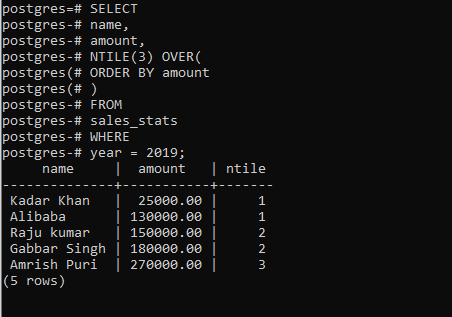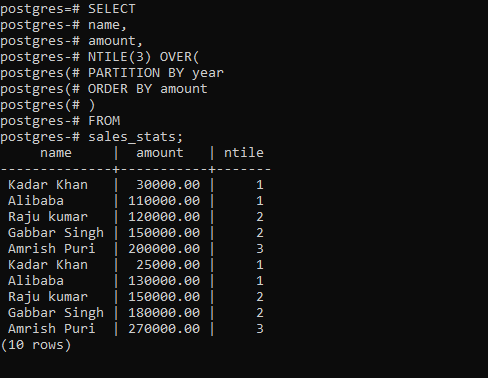# PostgreSQL – NTILE Function

• Last Updated : 08 Oct, 2021

In PostgreSQL, the NTILE() function is used to divide ordered rows in the partition into a specified number of ranked buckets. Buckets are nothing but ranked groups.

The syntax of the NTILE() looks like below:

```Syntax:
NTILE(buckets) OVER (
[PARTITION BY partition_expression, ... ]
[ORDER BY sort_expression [ASC | DESC], ...]
)```

Let’s analyze the above syntax:

• The buckets are the number of ranked groups. It can either be a number or an expression but the expression must evaluate a positive integer value.
• The PARTITION BY is an optional clause distributes rows into partitions.
• The ORDER BY clause is used to sort rows in each partition.

Example 1:

First, create a table named sales_stats that stores the  sales revenue by employees:

```CREATE TABLE sales_stats(
name VARCHAR(100) NOT NULL,
year SMALLINT NOT NULL CHECK (year > 0),
amount DECIMAL(10, 2) CHECK (amount >= 0),
PRIMARY KEY (name, year)
);```

Second, insert some rows into the sales_stats table:

```INSERT INTO
sales_stats(name, year, amount)
VALUES
('Raju kumar', 2018, 120000),
('Alibaba', 2018, 110000),
('Gabbar Singh', 2018, 150000),
('Amrish Puri', 2018, 200000),
('Raju kumar', 2019, 150000),
('Alibaba', 2019, 130000),
('Gabbar Singh', 2019, 180000),
('Amrish Puri', 2019, 270000);```

The below statement uses the NTILE() function to distribute rows into 3 buckets:

```SELECT
name,
amount,
NTILE(3) OVER(
ORDER BY amount
)
FROM
sales_stats
WHERE
year = 2019;```

Output:Example 2:

The below query uses the NTILE() function to divide rows in the sales_stats table into two partitions and 3 buckets for each:

```SELECT
name,
amount,
NTILE(3) OVER(
PARTITION BY year
ORDER BY amount
)
FROM
sales_stats;```

Output:My Personal Notes arrow_drop_up